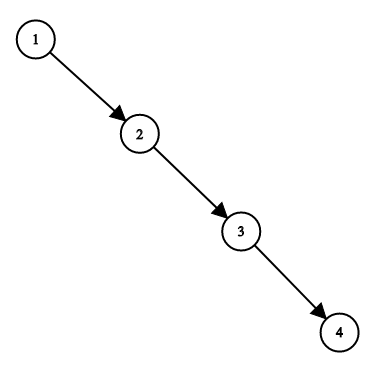# leetcode 1382. Balance a Binary Search Tree（python）

### 描述

Given a binary search tree, return a balanced binary search tree with the same node values.

A binary search tree is balanced if and only if the depth of the two subtrees of every node never differ by more than 1.

If there is more than one answer, return any of them.

Example 1:``````Input: root = [1,null,2,null,3,null,4,null,null]
Output: [2,1,3,null,null,null,4]
Explanation: This is not the only correct answer, [3,1,4,null,2,null,null] is also correct.

Note:

``````The number of nodes in the tree is between 1 and 10^4.
The tree nodes will have distinct values between 1 and 10^5.

### 解答

``````class Solution(object):
def balanceBST(self, root):
"""
:type root: TreeNode
:rtype: TreeNode
"""
L = []
def toList(root):
if not root:
return
toList(root.left)
L.append(root)
toList(root.right)
toList(root)
def toBiTree(order):
if not order:
return None
mid = len(order)//2
root = order[mid]
root.left = toBiTree(order[:mid])
root.right = toBiTree(order[mid+1:])
return root

### 运行结果

``````Runtime: 316 ms, faster than 95.15% of Python online submissions for Balance a Binary Search Tree.
Memory Usage: 21.9 MB, less than 90.78% of Python online submissions for Balance a Binary Search Tree.